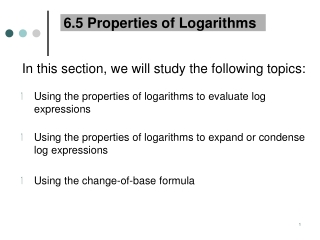DownloadDownload Presentation6.5 Properties of Logarithms

# 6.5 Properties of Logarithms

Download Presentation## 6.5 Properties of Logarithms

- - - - - - - - - - - - - - - - - - - - - - - - - - - E N D - - - - - - - - - - - - - - - - - - - - - - - - - - -
##### Presentation Transcript

1. 6.5 Properties of Logarithms In this section, we will study the following topics: Using the properties of logarithms to evaluate log expressions Using the properties of logarithms to expand or condense log expressions Using the change-of-base formula

2. Properties of Logarithmic Functions To solve various types of problems involving logarithms, we can make use of the following properties of logarithms.

3. Properties of Logarithms The four properties of logarithms listed earlier hold true for natural logarithms as well.

4. Examples • Find the exact value of each expression without using a calculator.

5. Other Properties of Logarithms

6. Properties of Logarithms WARNING!!!!!!

7. Properties of Logarithms ANOTHER WARNING!!!!!!

8. Using the Properties of Logarithms to Expand Log Expressions Use the properties of logs to write the expression as the sum and/or difference of logarithms (EXPAND).

9. Using the Properties of Logarithms to Expand Log Expressions

10. Using the Properties of Logarithms to Expand Log Expressions

11. Using the Properties of Logarithms to Expand Log Expressions

12. Using the Properties of Logarithms to Condense Log Expressions Use the properties of logs to write each expressions as a single logarithm (CONDENSE).

13. Change-of-Base Formula • I mentioned in a previous section that the calculator only has two types of log keys: • COMMON LOG (BASE 10) • NATURAL LOG (BASE e). • It’s true that these two types of logarithms are used most often, but sometimes we want to evaluate logarithms with bases other than 10 or e.

14. Change-of-Base Formula To do this on the calculator, we use a CHANGE OF BASE FORMULA. We will convert the logarithm with base a into an equivalent expression involving common logarithms or natural logarithms.

15. Change-of-Base Formula

16. Change-of-Base Formula Examples Example*: Use the change-of-base formula to evaluate log7264 using common logarithms using natural logarithms. Solution: The result is the same whether you use the common log or the natural log.

17. Change-of-Base Formula Examples Example: Use the change-of-base formula to evaluate: a) b)

18. End of Section 6.5